Sun, S., Mathias, J. D., Toussaint, E., and Grédiac, M. (2014). "Characterizing the variance of mechanical properties of sunflower bark for biocomposite applications," BioRes. 9(1), 922-937.

#### Abstract

Characterizing the variance of material properties of natural fibers is of growing concern due to a wide range of new engineering applications when utilizing these natural fibers. The aim of this study was to evaluate the variance of the Young’s modulus of sunflower bark by (i) determining its statistical probability distribution, (ii) investigating its relationship with relative humidity, and (iii) characterizing its relationship with the specimen extraction location. To this end, specimens were extracted at three different locations along the stems. They were also preconditioned in three different rel­ative humidity environments. The c2-test was used for hypothesis testing with normal, Weibull, and log-normal distributions. Results show that the Young’s modulus follows a normal distribution. Two-sample t-test results reveal that the Young’s modulus of sunflower stem bark strongly depends on the conditioning’s relative humidity and the specimen’s extraction location; it significantly decreased as the relative humidity increased and significantly increased from the bottom to the top of the stem. The correlation coefficients between the Young’s modulus of different relative humid­ity values and of specimen extraction locations were determined. The calculation of correlation coefficients shows a linear relation between the Young’s modulus and the relative humidity for a given location.

Characterizing the Variance of Mechanical Properties of Sunflower Bark for Biocomposite Applications

Shengnan Sun,a,b Jean-Denis Mathias,c,* Evelyne Toussaint,a,band Michel Grédiac a,b

Characterizing the variance of material properties of natural fibers is of growing concern due to a wide range of new engineering applications when utilizing these natural fibers. The aim of this study was to evaluate the variance of the Young’s modulus of sunflower bark by (i) determining its statistical probability distribution, (ii) investigating its relationship with relative humidity, and (iii) characterizing its relationship with the specimen extraction location. To this end, specimens were extracted at three different locations along the stems. They were also preconditioned in three different relative humidity environments. The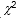-test was used for hypothesis testing with normal, Weibull, and log-normal distributions. Results show that the Young’s modulus follows a normal distribution. Two-sample t-test results reveal that the Young’s modulus of sunflower stem bark strongly depends on the conditioning’s relative humidity and the specimen’s extraction location; it significantly decreased as the relative humidity increased and significantly increased from the bottom to the top of the stem. The correlation coefficients between the Young’s modulus of different relative humidity values and of specimen extraction locations were determined. The calculation of correlation coefficients shows a linear relation between the Young’s modulus and the relative humidity for a given location.

Keywords: Sunflower bark; Mechanical testing; Young’s modulus; Statistical analysis; Relative humidity; Specimen extraction location

Contact information: a: Institut Pascal, Clermont Université, Université Blaise Pascal, BP 10448, F-63000 Clermont-Ferrand, France; b: CNRS, UMR 6602, Institut Pascal, 63177 Aubière Cedex, France; c: IRSTEA, Laboratoire d’Ingénierie pour les Systèmes Complexes, 9 Avenue Blaise Pascal, CS20085, 63178 Aubière, France; *Corresponding author: jean-denis.mathias@irstea.fr

INTRODUCTION

In recent decades, the use of natural fibers such as wood, hemp, ﬂax, jute, and sisal in composites has substantially increased due to growing environmental concerns; these fibers exhibit good qualities in terms of environmental safety, recyclability, low cost, and mechanical properties. These natural fibers can be classified in two groups depending on their production: primary plants and secondary plants. Primary plants are those grown for fiber production and to which agricultural fields are dedicated. Secondary plants produce a primary product, and their by-products are used as natural fibers. Agricultural by-products such as wheat straw, rice straw, and corn pith exhibit environmental compatibility. Some of these agricultural products possess qualities similar to those of natural fibers from primary plants, and they are generally cheaper. They are therefore being used in different biocomposite applications (Kargarfard and Latibari 2011; Panthapulakkal et al. 2006; Sun et al. 2013; Wang and Sun 2002; White and Ansell 1983; Yang et al. 2003).

Boards made of rice husk and sawdust exhibit good material properties, such as mechanical strength and water resistance, as well as noise and thermal insulation, with a competitive price when compared to wood board. Yang et al. (2003) reported that the noise and thermal insulation properties of rice straw have led to its substitution for wood in particle board and insulation board in wooden constructions. Viswanathan and Gothandapani (1999) produced and tested ceiling boards from agricultural rice husk and sawdust waste. They found that the produced sheets showed similar physical properties to those of commercial samples with respect to moisture content, water absorption rate, and mechanical strength. These advantages make agricultural by-products extremely competitive for use in composites, especially for industrial applications that require a compromise between mechanical properties, environmental compatibility, and cost.

We focus here on a promising agricultural by-product, sunflower stems. In Europe, sunflowers are widely cultivated for the edible oil extracted from their grains; however, there is no significant industrial use of the sunflower stems, which are shredded after the flowers are harvested. In a dry stem, the density of bark is 0.35, which is about 16 times greater than the pith. Considering a set of ten stems, it has been observed that the weight of the bark is equal to 89±33 grams. This corresponds to 94% of the total mass of the stem. The abundant sources (FAOSTAT 2010) and favorable mechanical properties of the bark (Sun et al.2013) make it possible to use this by-product for bio-sourced composite materials. Nevertheless, a main drawback is the lack of its reliability due to the large variance of its properties. Hence, characterizing the variance of its mechanical properties is necessary for future biocomposite applications.

The variance of several natural fiber properties has been studied in the literature. The first study concerns the statistical analysis of fiber strength distribution and fiber dimension aspect distribution using symmetrical Gaussian and non-symmetrical Weibull / log-normal distributions. Zafeiropoulos et al. (2007) highlighted the effect of surface treatment on ﬂax fiber strength by the analysis of variance (ANOVA) based on the assumption that fiber strength follows a normal distribution. The surface treatment effect was also studied using a Weibull distribution (Zafeiropoulos and Baillie 2007). However the ANOVA method cannot be used in this latter case; thus, the significance analysis is performed only by observing whether the mean values are within confidence intervals. Both methods find that surface treatment does not significantly change fiber strength. The elementary flax fiber studies of Joﬀe et al. (2003) and Andersons et al. (2005) revealed that fiber strength is reasonably well-approximated by the two-parameter Weibull distribution. Distribution parameters were determined using the maximum likelihood method. For wheat straw fibers, a good ﬁt was observed between fiber strength and the two-parameter Weibull distribution (Panthapulakkal et al. 2006). The dimension aspect distribution of wood pulp fibers was studied by Pulkkinen et al. (2006) with normal, log-normal, Weibull, and gamma distributions. Among these, Weibull distribution was found to ﬁt the experimental distributions. Yao et al. (2008) found that fiber length and aspect ratio distributions for all fibers in rice straw fiber-reinforced composite followed a log-normal distribution.

The second type of study on variance concerns the analysis of physical and structural property relationships (Charlet et al.2010). The conclusion is that variance in the mechanical properties of ﬂax fiber may be primarily due to the variation in the cellulose content from one fiber to another, and also to the randomness of the location and size of defects along each fiber. Concerning sunflower stem properties, it was found in a previous study (Sun et al. 2013) that the variance of the Young’s modulus of sunflower bark specimens is not only due to natural variability in plants, but may also be due to the conditioning’s relative humidity and to the specimen extraction location along the stem. However, the sample size was not large enough to conduct a statistical test and to give confidence in the results.

Consequently, 810 mechanical tests were conducted in the present study to provide a sufficient number of results to study the variance of the Young’s modulus. In the first part, we describe the mechanical experimental set-up, including specimen preparation. In the second part, we present the statistical tools used in this study: statistical distribution histograms of Young’s modulus,-test, two-sample t-test, and correlation coefficients. In the third part, both the statistical distribution of the Young’s modulus and the influences of relative humidity and specimen extraction location on the Young’s modulus are investigated using statistical tools (significance tests and correlation coefficients) applied to our experimental results.

EXPERIMENTAL

Specimen Preparation and Mechanical Tests

Bark specimens were extracted from different stem locations and conditioned at different relative humidity (RH) conditions before each test to evaluate the influence of these parameters on the Young’s modulus of the bark.

Bark specimens

The sunflower species used for this study was LG 5474 (Limagrain Verneuil Holding LVH), grown in Perrier, France, in 2010. The locations on the sunflower stem where bark specimens were extracted are presented in Fig. 1. Stems were selected that were 765 mm in length. One end of the stem was the location of the first stem node above the roots. From this end of the stem, three different height sections (bottom, middle, and top) that were 75 mm in length were cut out to obtain bark specimens. Using these three sections enables the influence of the specimen extraction location on the Young’s modulus to be evaluated. Each section was longitudinally divided (along the fiber direction) into six parts (see cross section A-A in Fig. 1).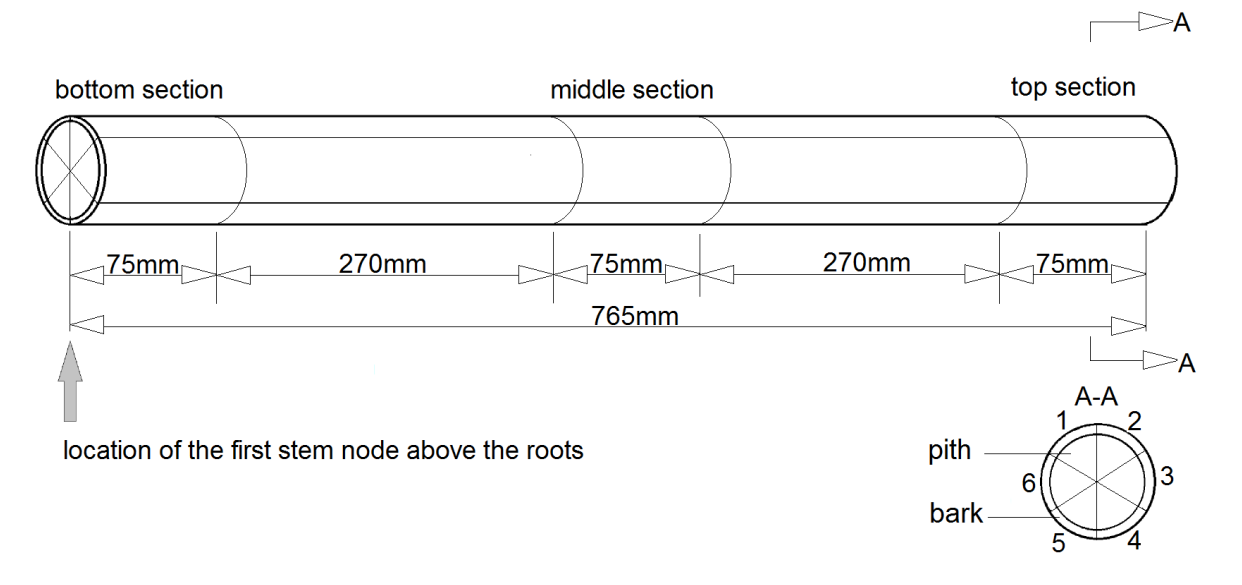Fig. 1. Specimen extraction locations

These six parts were processed to obtain rectangular bark specimens. For this, the inner part of the bark was carefully polished with sandpaper to remove the protruding material (i.e.,xylem-type tissue). This process did not influence the test result because the quantity of xylem-type tissue removed was small when compared to the quantity of bark. In this study, 270 bark specimens were obtained to provide sufficient data for statistical tests. They were cut from 15 different stems. This means that there were 90 specimens at each specific height location, which accounted for one-third of the total quantity. Each specimen was reconditioned at a different RH before each mechanical test.

Humidity conditioning

Specimens were conditioned in chambers at different RH values before testing to evaluate the influence of the specimen’s moisture content on the Young’s modulus. The RH conditioning values used for this study were 0% RH, 33% RH, and 75% RH. The conditioning chamber at 0% RH was an oven set at 60 °C and equipped with P2O5 desiccant. The 33% RH and 75% RH conditioning chambers were constructed using the method described in ISO Standard 483:2006 (ISO 483, 2006), i.e., different saturated aqueous solutions were placed in the chambers: saturated solution of magnesium chloride for 33% RH; and saturated solution of sodium chloride for 75% RH. The temperature in the chambers was equal to the room temperature. According to the experimental observations obtained in a previous study (Sun et al. 2013), specimens were kept in the chamber for at least three days to reach moisture equilibrium. The room temperature at the end of the conditioning stage was 20 ± 2 °C.

Mechanical tests

Tensile tests were carried out on a Deben MICROTEST 2KN testing machine (Deben UK Ltd., Edmunds, Suffolk, UK), which is suitable for testing small specimens. The machine was equipped with a 2-kN load cell. The cross-head displacement rate during the tests was equal to 2 mm/min. The clamping length was 30 mm. Specimens were carefully clamped to prevent fiber damage and to ensure that the clamping force was sufficient to avoid any test slippage. The room temperature during the mechanical tests was 20 ± 2 °C.

Statistical Analysis Methods

As noted above, the experimental results obtained in the study of Sun et al. (2013) exhibited significant variability. We therefore proceeded to characterize this variance using suitable statistical tools. The probability distribution function was first studied to determine the variance properties of the Young’s modulus obtained at each RH and specimen extraction location. Knowing the probability distribution function is also important for choosing the correct statistical method for significance testing. The RH value and specimen extraction location were examined to determine if they had a significant influence on the Young’s modulus. Finally, correlation coefficients were calculated to determine the potential statistical dependency of RH and extraction location on the Young’s modulus.

Probability distribution test

Histograms were used to determine some assumptions concerning the probability distribution of the Young’s modulus of sunflower bark. A statistical hypothesis test was then performed to determine the distribution followed by the sunflower bark.

I) Statistical histograms

In statistical analysis, the histogram, one of the most relevant graphs, visually and directly presents the frequency distribution of a dataset (Chiang 2003). Hence, the frequency distribution histograms of the Young’s modulus were plotted. These distribu-tion histograms were used to model the probability distribution function of the data. Statistical hypothesis testing, described in the following section (“Statistical hypothesis testing of probability distributions”), was performed on these datasets with the various probability distribution functions estimated from the histograms. The selection of the bin number (or bin size) is important for the representation and the description of histograms. A large bin number may generate perturbations, while a small bin number may lead to a loss of information. Previous methods for determining the bin numbers (Sturges 1926; Scott 1979) have these disadvantages. In the present work, we propose a method that has recently been suggested by Shimazaki and Shinomoto (2007). In the cited paper, the optimal bin number is determined by minimizing an estimated cost function obtained from a modified mean integrated square error (MISE) method. The MISE supplies the error between the estimated probability density represented by the histogram and the actual (and unknown) probability of a dataset.

II) Testing statistical hypothesis of probability distributions

Once the statistical data had been obtained, it was necessary to determine the probability distribution. For this purpose, we used statistical hypothesis testing on the experimental Young’s modulus distribution of the bark. The hypothesis testing for the probability distribution consists of the following steps:

• Stating a null hypothesis H0: The probability distribution of the Young’s modulus, , of the sunflower bark extracted at a certain location and preconditioned at a certain RH follows either a normal, log-normal, or Weibull distribution.

• Deciding whether to reject or accept H0: The decision is often taken with a probability threshold,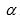, which is called the significance level. If the p-value<, then His rejected and the difference between the probability distribution of the and the currently tested distribution is significant. If the p-value>, then His accepted, and the difference between the probability distribution of the and the currently tested distribution is not significant. This means that we cannot reject the hypothesis that the data follow the current tested distribution function. Thevalue is conventionally chosen to be 0.05 because this is the critical point between weak and strong evidence against H0, as described by Wasserman (2004). The p-value is the probability of wrongly rejecting Hif it is in fact true. Thus, the p-value is also a measure of the evidence against H0.

Therefore, the smaller the p-value is, the stronger the evidence against H0:

• p-value<0.01: very strong evidence against H0;
• 0.01<p-value<0.05: strong evidence against H0;
• 0.05<p-value<0.1: weak evidence against H0;
• p-value>0.1: little or no evidence against H0.

In the present work, the-test was conducted for hypothesis testing using the chi-squared goodness-of-fit function, chi2gof, in the statistical toolbox of the MATLAB software program (MATLAB 2009). Using this function enabled us to obtain the p-value to perform hypothesis testing. The-test was performed by assembling the data into bins, calculating the observed and expected counts for these bins, and computing the-test. The expected counts should be at least 5 to validate theapproximation. The-test is sensitive to the choice of bins. There is no optimal choice for the bin width for the-test; however, reasonable choices should produce similar results. The chi2gof function of MATLAB sets the number of bins to 10 by default, and bins with an expected count less than 5 are pooled with neighboring bins until the count in each extreme bin is at least 5. The-test equation is,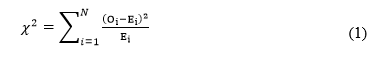where Oi are the observed counts in each bins concerned by the tested data, Ei is the expected count in each bin corresponding to the current tested probability distribution, and N is the number of bins. The corresponding p-value is found in the statistical-test table.

Significance test

The purpose of the significance test is to evaluate the significance of the differences in the mean values of the Young’s modulus obtained at various locations and RH values. This allows us to determine if the specimen extraction location and the moisture content of the specimens influence the Young’s modulus. Testing methods depend on the normality of the data. The two-sample Student t-test is the most commonly used method to evaluate the differences in the means of two datasets. To use the t-test, the datasets must exhibit a normal distribution. As described earlier, the normality assumption can be evaluated by observing the data distribution (via histograms) and by performing a normality hypothesis test. The mean value of a dataset is denoted as mean (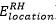). The two-sample t-test procedure consists of the following steps (Wasserman 2004):

• Establishing the null hypothesis H0: For example, mean(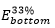) = mean(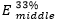) is denoted as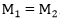.

• Applying the statistical test: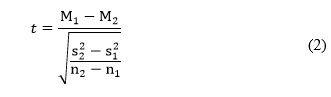where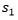and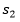are the dataset standard deviations and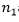and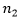are the dataset sample sizes.

• Finding the p-value in the Student t distribution table.

• Comparing thevalue (e.g.= 0.05) and the p-value: As has been described earlier in the previous section (“Probability distribution test”). Here the difference between mean() and mean() is significant when the p-value<. This test can be performed using the two-sample t-test function, ttest2, in the statistical toolbox of the MATLAB software program (MATLAB 2009).

In the case of non-normality of the data, the Mann-Whitney Utest (a non-parametric test of the H0) can be performed in the place of the two-sample t-test (Sijtsma and Emons 2010). The main advantage of the U test is it does not require a normally distributed data set. The U test method is different for small and large samples (,>20). Because the present study has a large sample size, the determined value in the test is assumed to be normally distributed. Taking the Hof mean() = mean() as an example, the test procedure is as follows:

• Arranging the data: Arranging all the data of the two datasetsandinto a single ranked series, then adding up the ranks of the data that come from the datasetand.

The sums are denoted as R1 and R2, and they have the relationship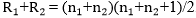.

• Determining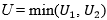with: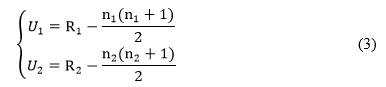• Calculating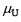and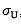, the mean and standard deviation of U, respectively: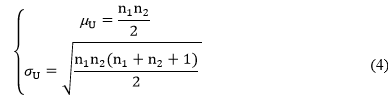• Calculating the standard normal statistic: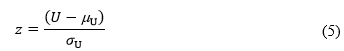• Determining the p-value from the statistical z-table (normal distribution): The decision rule here is the same as that described for the two-sample t-test. This test can be carried out using the Wilcoxon rank sum test function, ranksum, in the statistical toolbox of the MATLAB software program (MATLAB 2009).

Correlation coefficient between different testing conditions

Specimens were expected to show a statistical dependence according to their extraction location and the RH. This dependence may be estimated by calculating the correlation coefficient. The correlation coefficient represents the strength of a linear relationship between the variables (in this study, the specimen extraction locations and the RH). It is calculated with the correlation coefficients function, corrcoef, of the MATLAB software program (MATLAB 2009). DATA is an N×2 matrix in which the rows are the observations and the columns are the two different testing conditions (e.g., different RH for the same specimen extraction location, or different specimen extraction locations for the same RH). N is the number of specimens for which the testing results are used. It is smaller than 90 because in the present study some specimens were damaged during the cycle of the tests. The correlation coefficient R(i,j) of the 2×2 symmetric correlation matrix R is calculated as follows: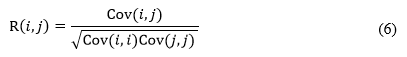where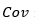is the covariance matrix of DAT A, and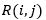is the correlation coefficient between two testing conditions that correspond to row i and column j in the R matrix.

RESULTS AND DISCUSSION

Mechanical Test Results

The Young’s modulus of the sunflower bark tested at different specimen extraction locations and RH is now discussed. The box plot of the experimental results is presented in Fig. 2. Two trends can be observed: the Young’s modulus increased as the specimen extraction height increased, and it decreased as the RH or the specimen moisture content increased. However, the high standard deviations make it difficult to confirm these trends. A significance test was therefore performed to evaluate the significance of the difference between these results (see “Influence of RH and specimen extraction location”) from the probability distributions determined in “Probability distribution”. In each case, the correlation relationship between the Young’s modulus obtained for different RH and the specimen extraction location is determined. The results obtained are presented and discussed in “Young’s modulus correlation coefficient between different testing conditions”.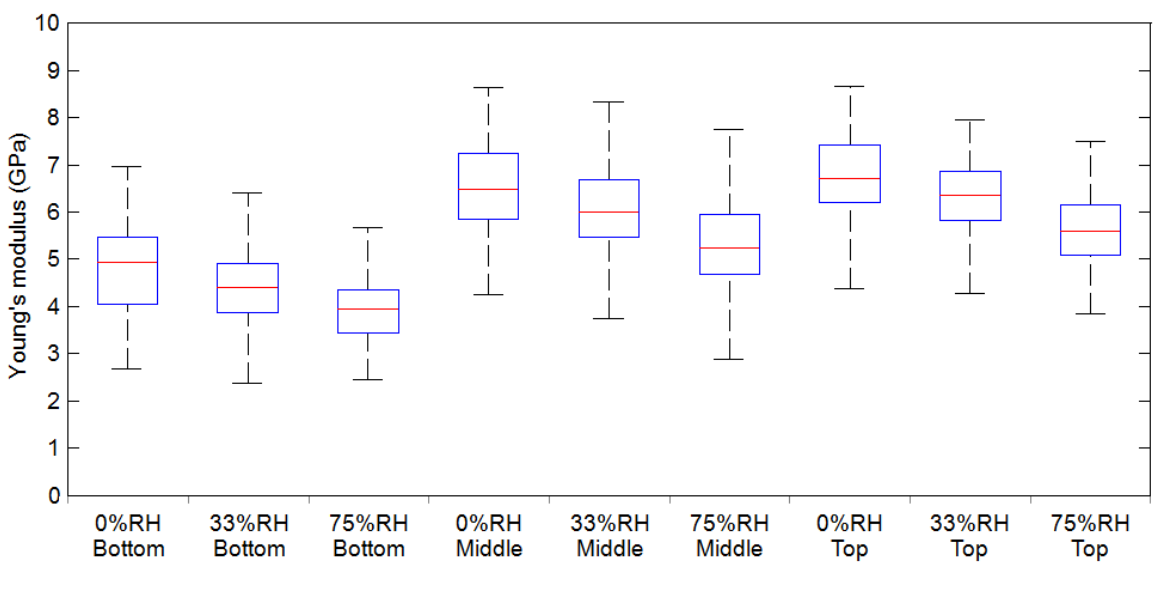Fig. 2. Box plot of the Young’s modulus of bark

Probability distribution function

Figure 3 represents a typical histogram and probability function plots. The plotted dataset is for the specimens at 0% RH for the bottom location. In Fig. 3-(a), the fitted normal, Weibull, and log-normal distributions are superimposed. The empirical normalized cumulative distribution functions (CDF) for these three distributions are presented in Fig. 3-(b)-(c)-(d). Both the histogram and the empirical CDF plots were in good agreement with the fitted normal, Weibull, and log-normal distributions. Thus, the nine datasets in Fig. 2 may potentially follow normal, Weibull, and log-normal distributions. The objective now was to refine this first conclusion by performing hypothesis tests assuming either normal, Weibull, or log-normal distributions. It is worth recalling that (i) the null hypothesis cannot be rejected when the p-value is greater than 0.05, and (ii) the greater the p-value, the weaker the evidence against the null hypothesis (Wasserman 2004).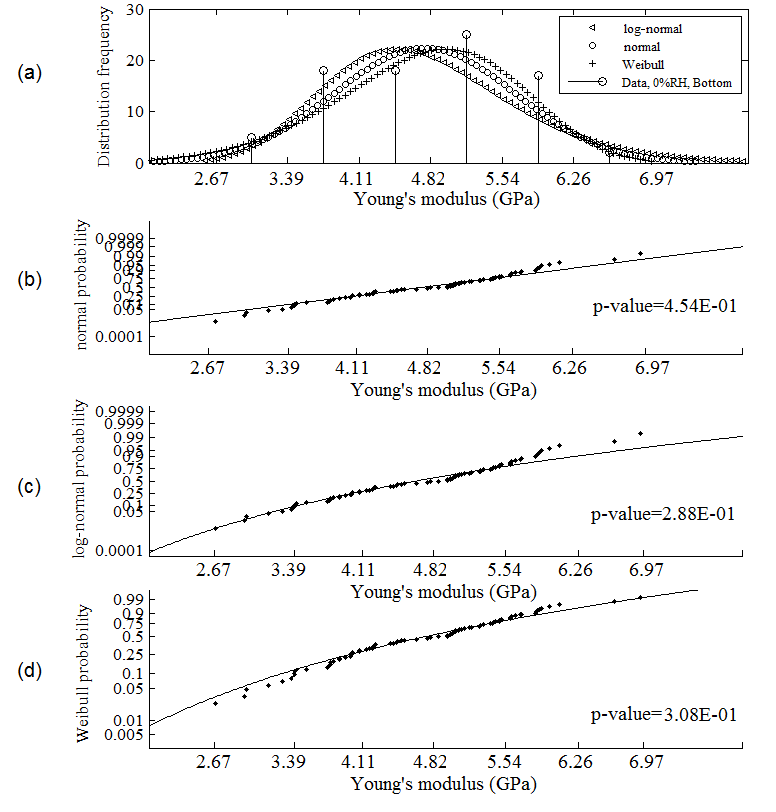Fig. 3. Histogram and probability plot at 0% RH and bottom location: (a) Histogram; (b) normal probability plot; (c) log-normal probability plot; and (d) Weibull probability plot

From the results of the distribution test presented in Fig. 4, it can be said that all the p-values were greater than 0.1. The only exception to this observation was for the 0% RH-top dataset assuming a Weibull distribution; in this case, the p-value was equal to 0.018 and thus much smaller than 0.05. This is because the observed counts were significantly lower than the expected total counts for the Young’s modulus range of 4.37 to 6.09 GPa and the observed counts were greater for the range of 6.09 to 6.95 GPa. This observation can be verified by examining the details of the test results. In conclusion, the normal and the log-normal distributions are followed by all nine datasets. This conclusion is obtained at a 95% significance level, where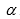is equal to 0.05 (remembering thatcorresponds to the value from which H0 is accepted or rejected); thus, we can examine whether one of these two distributions is more representative of the datasets by comparing the p-values obtained for each of them. Among these nine datasets, six of them corresponded to a situation where the p-value of the normal distribution test was the highest, whereas the remaining three corresponded to a situation where the log-normal distribution was the highest. The p-value of the normal distribution function is, therefore in most cases, greater than that of both the Weibull and the log-normal distribution functions. This indicates that the evidence againstthe null hypothesis that the datasets follow a normal distribution is the weakest. Therefore, the final conclusion is that the datasets are more inclined to follow a normal distribution function. The two-tailed t-test was therefore only performed for the normal distribution function to determine the influence of both the RH and the specimen extraction location. This is discussed in the following section.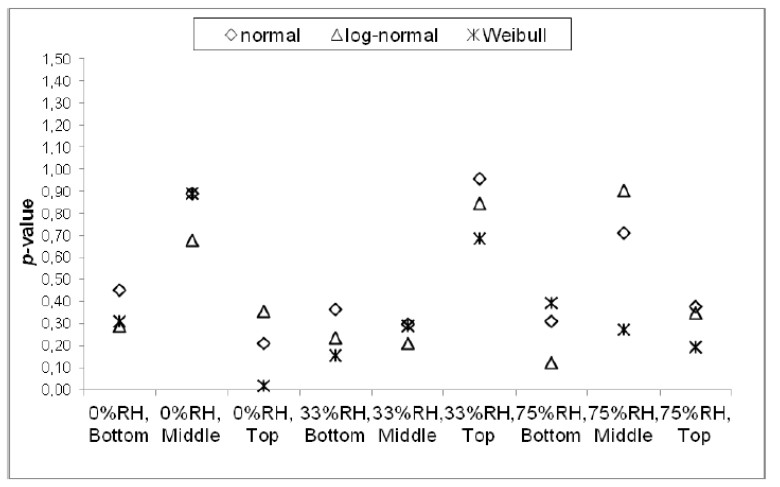Fig. 4. p-values for all distribution tests

Influence of RH and specimen extraction location

The influence of both the RH and the specimen extraction location is now analyzed. The p-values obtained from the two-sample t-test performed on the nine datasets are given in Fig. 5. It can be seen that the smaller the p-value, the stronger the evidence against the null hypothesis. p-values were less than 0.035 for all the test results. This indicated that the differences between the mean values of the datasets were significant for= 0.05. Concerning the influence of the specimen extraction location, it can be concluded from Fig. 5 that the differences between the middle and top locations for the three RH values were less significant than those between the bottom and middle locations. Indeed, the p-value of the former was the highest: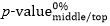=2.71×10-2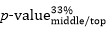= 3.44×10-2,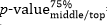=6.90×10-3, and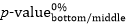,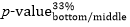and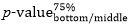< 10-16. Moreover, for the two-sample t-test between the middle and the top locations, the p-value was the smallest at 75% RH. Consequently, the difference between the Young’s modulus obtained at the middle and the top locations was the most significant.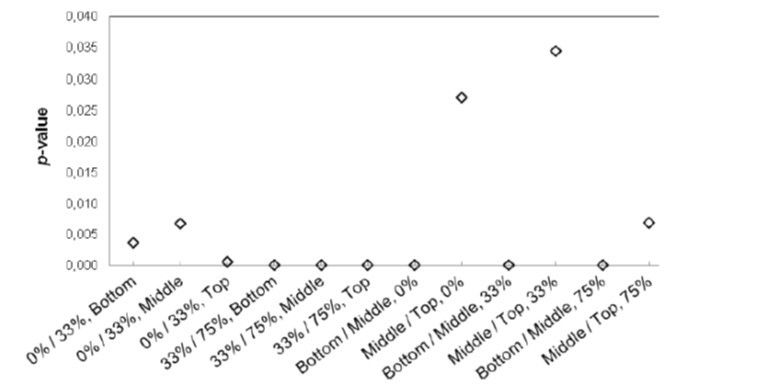Fig. 5. p-values for the two-sample t-test

For the influence of RH, it can be observed at the same location that the p-value of the two-sample t-tests between 0% RH and 33% RH was always greater than that obtained between 33% RH and 75% RH. Hence, the difference between 33% RH and 75% RH is the greatest. In conclusion, both the specimen moisture content and the specimen extraction location have a significant influence on the Young’s modulus when=0.05. Hence, the Young’s modulus significantly decreased with an increase of the specimen’s RH conditioning. This confirmed the trend observed in our previous work (Sun et al. 2013). In the latter case, it was due to water softening the cell wall of the fiber (Li 2006). Furthermore, the decrease was more significant when the RH value increased from 33% to 75%. This was probably because the difference between 33% RH and 75% RH is greater. It can also be said that the Young’s modulus of the sunflower bark tended to increase with specimen extraction location along the stem. This increase was greater from the bottom to the middle than from the middle to the top. This confirmed our earlier conclusion (Sun et al. 2013): the increase of the Young’s modulus of sunflower bark with specimen extraction location along the stems was due to the increase in the sclerenchyma content, which is a rigid material and is more visible between the bottom and the middle. This observation of Young’s modulus was also due to the decrease in the porosity of the bark along the stem.

Young’s modulus correlation coefficient between different testing conditions

Some relevant correlation coefficients extracted from the correlation matrices are presented in Tables 1 and 2. In Table 1, the correlation that existed between the Young’s modulus and the RH value is clearly evidenced for each specimen extraction location, as exemplified by the high correlation coefficient obtained in each case. In Table 2, the correlation coefficients were much lower than in the previous case. This indicated that the correlation between the Young’s modulus and the specimen extraction location was much lower than the correlation between the Young’s modulus and the RH value.

Table 1. Correlation Coefficients between Different RH Values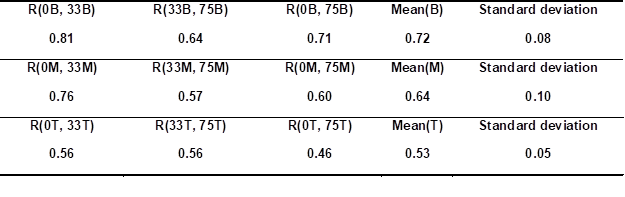0, 33, and 75 are 0%, 33%, and 75% RH, respectively; B, M, and T are bottom, middle, and top, respectively

Table 2. Correlation Coefficients between Different Specimen Extraction Locations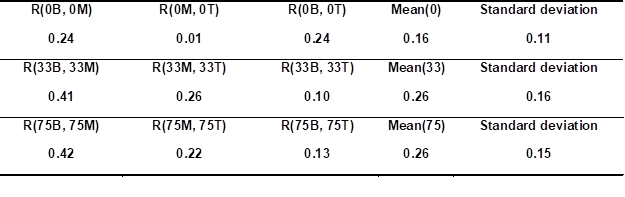0, 33, and 75 are 0%, 33%, and 75% RH, respectively; B, M, and T are bottom, middle, and top, respectively

This difference between correlation coefficients obtained in each case can be partially explained as follows. In the first case, the Young’s modulus is obtained with the same specimens for various RH values. In the second case, however, the tested specimens are not the same because they are extracted from different stems, which can have, for instance, different maturation and growth conditions along the stem. These differences potentially induce greater variability, which partially contributes to the lower correlation coefficients. A typical correlation curve of the Young’s modulus at 0% and 33% RH is presented in Fig. 6. The linear dependence is clearly visible.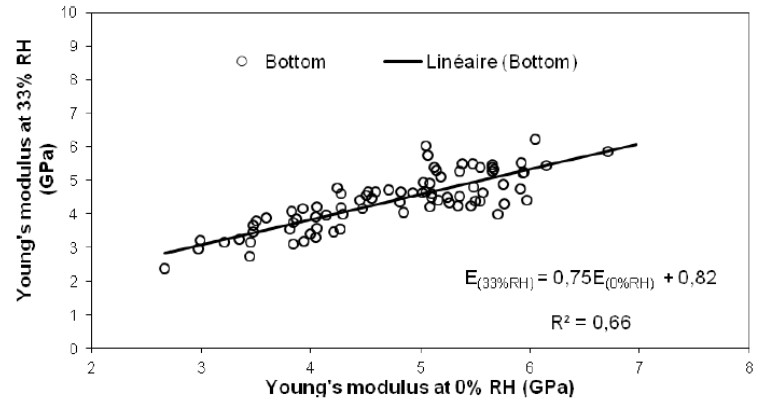Fig. 6. Correlation relationship between 0% and 33% RH at the bottom specimen extraction location

Similar figures can be obtained for the other RH values and locations (not shown). In each case, a linear regression equation was obtained. These equations are shown in Table 3.

Table 3. Linear Regression of the Young’s Modulus between Different RH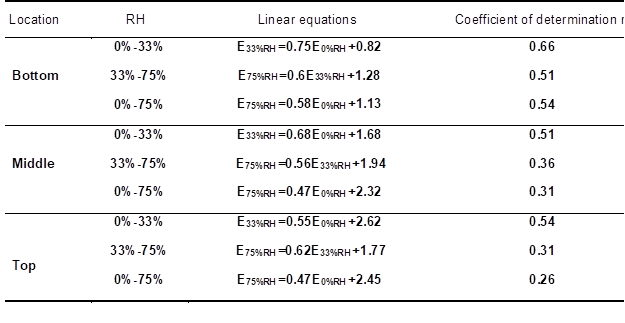The average value of the slope of the nine corresponding lines was 0.59 (with a standard deviation equal to 0.09). The slope values tended to decrease with increasing RH between the different RH values (see column 2) and with increasing height of the specimen extraction location (see column 1). The linear regression equations representing the correlation between the Young’s modulus and the different specimen extraction locations are not presented here because, as explained above, these parameters were less strongly correlated, thus leading to determination coefficients that were very small in comparison with those reported in Table 3.

CONCLUSIONS

1. Hypothesis testing was performed on the datasets obtained in each case. Probability distribution tests showed that these datasets follow a normal distribution function.
2. Significance tests showed that the Young’s modulus closely depended on both the RH value and the specimen extraction location. This modulus tended to significantly increase from the bottom to the top of the stems and tended to significantly decrease with increasing RH. Statistically, the difference in Young’s modulus between the bottom and middle locations was more significant than the difference between the middle and the top locations at all RH values. Furthermore, the differences in the Young’s modulus between the middle and the top locations were greater for the higher RH values. The difference in Young’s modulus between 33% RH and 75% RH was greater than that between 0% RH and 33% RH. This was probably because the difference between 33% RH and 75% RH was greater.
3. The correlation coefficients between the Young’s modulus at various RH and specimen extraction locations were characterized. It was found that the correlation coefficient between the Young’s modulus and the RH value was greater than that obtained between the Young’s modulus and the specimen extraction location. Finally, the linear regression equations relating the Young’s modulus to the RH values were determined, which showed the dependence of the experimental results for a given extraction location.

ACKNOWLEDGMENTS

The authors would like to acknowledge the French National Research Agency (ANR), Céréales Vallée, and ViaMéca for their financial support (ANR-10-ECOT-004 Grant). The authors are also grateful to Mr. D. Zheng for his contribution to the experimental part of this study.

REFERENCES CITED

Andersons, J., Spārniņš, E., Joﬀe, R., and Wallström, L. (2005). “Strength distribution of elementary flax fibres,” Compos. Sci. Technol. 65(3-4), 693-702.

Charlet, K., Jernot, J., Breard, J., and Gomina, M. (2010). “Scattering of morphological and mechanical properties of ﬂax fibers,” Ind. Crops Prod. 32(3), 220-224.

Chiang, C. L. (2003). Statistical Methods of Analysis, World Scientific, Singapore.

FAOSTAT. (2010). “FAO statistics division 2010: Europe sunflower seed area harvested,” Food and Agriculture Organization (FAO) of the United Nations (http://faostat.fao.org/DesktopDefault.aspx?PageID=567).

ISO 483. (2006). “Plastics – Small enclosures for conditioning and testing using aqueous solutions to maintain the humidity at a constant value,” UNE-EN ISO 483:2006, International Organization for Standardization, Geneva, Switzerland.

Joﬀe, R., Andersons, J., and Wallström, L. (2003). “Strength and adhesion characteristics of elementary ﬂax fibres with different surface treatments,” Compos. Part A: Appl. Sci. Manuf.34(7), 603-612.

Kargarfard, A., and Latibari, A. J. (2011). “The performance of corn and cotton stalks for medium density fiberboard production,” BioResources 6(2), 1147-1157.

Li, D. (2006). Fiber Materials (Textile Higher Education Fifth Ministerial-Level Planning Materials)(Chinese Edition), China Textile & Apparel Press, Beijing.

MATLAB (2009). Computer software, R2009 version. (http://www.mathworks.com/ products/matlab/). Last accessed March 2012.

Panthapulakkal, S., Zereshkian, A. and Sain, M. (2006). “Preparation and characterization of wheat straw fibers for reinforcing application in injection molded thermoplastic composites,” Bioresource Technol. 97(2), 265-272.

Pulkkinen, I., Ala-Kaila, K., and Aittamaa, J. (2006). “Characterization of wood fibers using fiber property distributions,” Chem. Eng. Process. 45(7), 546-554.

Scott, D. (1979). “On optimal and data-based histograms,” Biometrika 66(3), 605-610.

Shimazaki, H., and Shinomoto, S. (2007). “A method for selecting the bin size of a time histogram,” Neural Comput.19(6), 1503-1527.

Sijtsma, K., and Emons, W. (2010). “Nonparametric statistical methods,” in: International Encyclopedia of Education, Third Ed., Vol. 7, P. Peterson, E. Baker, and B. McGaw (eds.), Elsevier, Oxford, pp. 347-353.

Sturges, H. (1926). “The choice of a class interval,” J. Am. Stat. Assoc. 21(153), 65-66.

Sun, S., Mathias, J. D., Toussaint, E., and Grédiac, M. (2013). “Hygromechanical characterization of sunflower stems,” Ind. Crops Prod. 46(4), 50-59.

Viswanathan, R., and Gothandapani, L. (1999). “Mechanical properties of coir pith particle board,” Bioresource Technol.67(1), 93-95.

Wang, D., and Sun, X. (2002). “Low density particleboard from wheat straw and corn pith,” Ind. Crops Prod. 15(1), 43-50.

Wasserman, L. (2004). All of Statistics: A Concise Course in Statistical Inference. Springer Verlag, New York.

White, N., and Ansell, M. (1983). “Straw-reinforced polyester composites,” J. Mat. Sci. 18(5), 1549-1556.

Yang, H., Kim, D., and Kim, H. (2003). “Rice straw-wood particle composite for sound absorbing wooden construction materials,” Bioresource Technol. 86(2), 117-121.

Yao, F., Wu, Q., Lei, Y., and Xu, Y. (2008). “Rice straw fiber-reinforced high-density polyethylene composite: Effect of fiber type and loading,” Ind. Crops Prod. 28(1), 63-72.

Zafeiropoulos, N., Dijon, G., and Baillie, C. (2007). “A study of the effect of surface treatments on the tensile strength of ﬂax fibres: Part I. Application of Gaussian statistics,” Compos. Part A: Appl. Sci. Manuf. 38(2), 621-628.

Zafeiropoulos, N., and Baillie, C. (2007). “A study of the effect of surface treatments on the tensile strength of ﬂax ﬁbres: Part II. Application of Weibull statistics,” Compos. Part A: Appl. Sci. Manuf. 38(2), 629-638.

Article submitted: October 10, 2013; Peer review completed: December 4, 2013; Revised version received: December 10, 2013; Accepted: December 17, 2013; Published: December 20, 2013.